# Particle Size Distribution Measurement by the Laser Diffraction/Scattering Method Part3

## Particle Size Distribution with Laser Diffracted/Scattered Light

### Measuring Particle Size Distributions Using the Light Intensity Distribution Data of Diffracted/Scattered Light

The following shows four examples of light intensity distribution data and particle size distributions measured (calculated) using these data.

(The examples shown below were obtained using the SALD-2100. If a different model of instrument is used, the light intensity distribution data obtained by measuring even the same sample will be different.)

### Example 1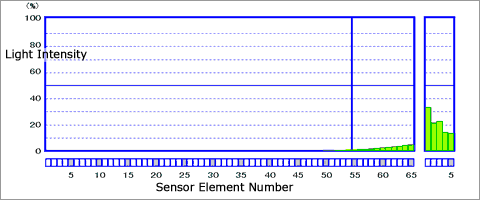Light Intensity Distribution Data of Diffracted/Scattered Light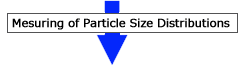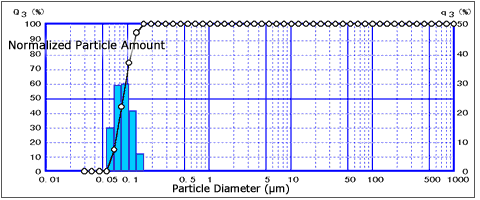Particle Size Distribution Data

### Example 2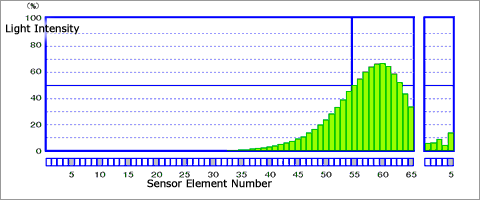Light Intensity Distribution Data of Diffracted/Scattered Light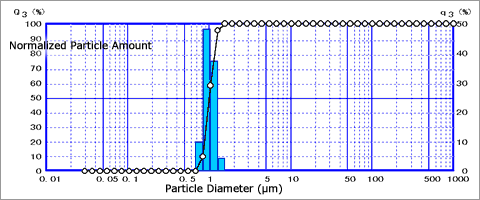Particle Size Distribution Data

### Example 3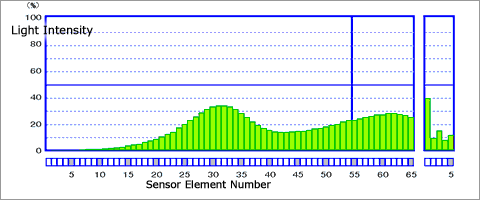Light Intensity Distribution Data of Diffracted/Scattered Light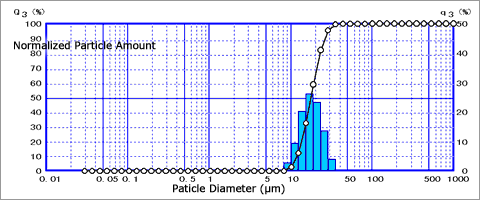Particle Size Distribution Data

### Example 4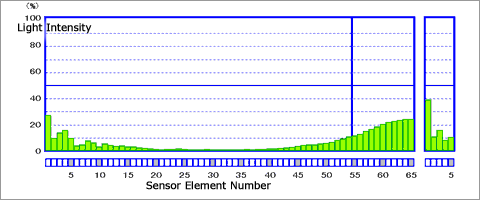Light Intensity Distribution Data of Diffracted/Scattered Light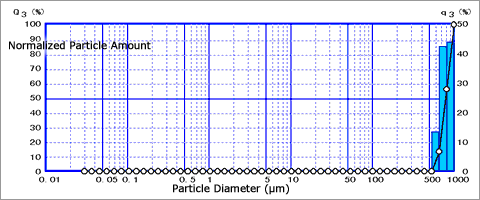Particle Size Distribution Data

Thus, a particle group is irradiated with laser light and the particle size distribution is calculated from the light intensity distribution data of diffracted/scattered light emitted from that particle group. I hope that you could see this process. With diffracted/scattered light, it is the pattern that is important NOT its intensity.